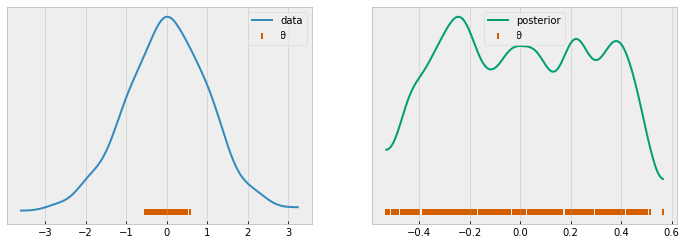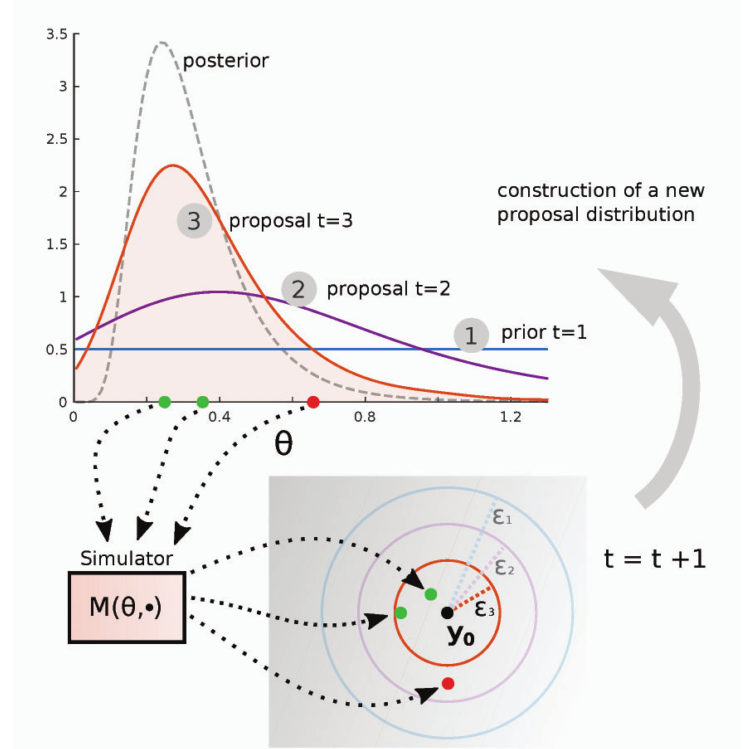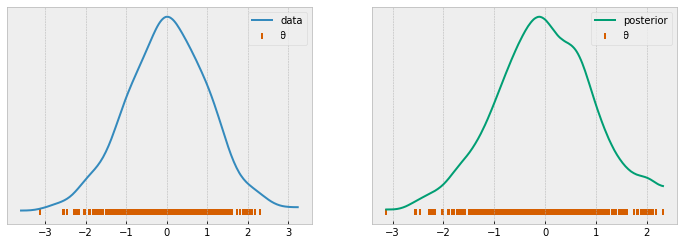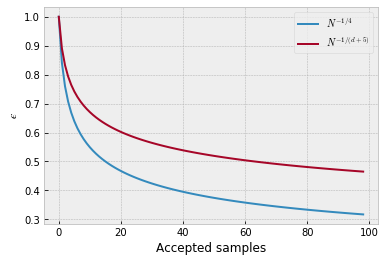Last friday I participated in PyMC3’s journal club meeting. I talked to PyMC3 members about my Google Summer of Code project. In this post I am showing the jupyter notebook I used to guide the talk. It was very helpful to get feedback from the developers about my project ideas. I look forward to join in next journal club meetings :).

# Developping a module for Approximate Bayesian Computation for PyMC3

Student: Agustina Arroyuelo

Mentors: Junpeng Lao, Osvaldo Martin

%matplotlib inline
import numpy as np
import pymc3 as pm
import matplotlib.pyplot as plt
import arviz as az
plt.style.use('bmh')


# What is ABC?

Approximate bayesian computation is a class of computational methods, also called Likelihood free inference methods, that bypass the evaluation of the likelihood function.(Sunnaker, 2013).

# Why do we need Approximate Bayesian Computation or Likelihood Free Inference?

We need ABC because for some models the likelihood function is very complex and it’s evaluation can be computationally costly. These models are typically called simulator based model. ABC is key in parameterizing simulator based models.

# What are Simulator Based Models? $M(\theta, V) = y$

• The likelihood function cannot be computed or is intractable due to their stochastic nature
• Typically have multiple parameters
• Given a set of parameters, it is ‘easy’ to generate data from them

# The most simple ABC algorithm is rejection ABC

# rejection ABC sampling
def rejection_abc(model=None, samples=1000, ϵ=0.5, plot=True):
"""
Rejection ABC sampling

Parameters
----------
model: pymc3 model
samples : int
Number of sampling steps
ϵ : int or float
Threshold value

Returns
-------
accepted : list
List of accepted θ
"""

model = pm.modelcontext(model)
prior = model.unobserved_RVs
data = model.observed_RVs.observations
size = len(data)
scale = data.std()
mean = data.mean()
accepted = []
ys = []

for _ in range(samples):
θ = prior.random() # prior sample
y_θ = np.random.normal(loc=θ, scale=scale, size=size) # simulator
ys.append(y_θ)
if np.absolute(mean - y_θ.mean()) < ϵ: # distance function, summary statistic
accepted.append(θ)

if plot:
_, ax = plt.subplots(1, 2, figsize=(12, 4))
az.kdeplot(data, label='data', ax=ax)
az.kdeplot(accepted, label='posterior', c='C7', ax=ax)
[ax[i].scatter(accepted, *len(accepted), marker='|', label='θ', c='C4') for i in range(len(ax))]
[ax[i].legend() for i in range(len(ax))]
[ax[i].set_yticks([]) for i in range(len(ax))]

return accepted, ys

data = np.random.randn(1000)
with pm.Model() as model:
a = pm.Normal('a')
l = pm.Normal('l', observed=data)

rej_abc = rejection_abc(model=model)# For likelihood free inference we need…

• A simulator based model
• To choose a Summary statistic or a vector of them
• A threshold
• A distance function

# SMC - ABC is a bit more complex…(Lintusaari, 2016)

1- $\theta$’s are drawn from the prior, if it is the first stage, or proposal distribution if it is not.

2- They are passed to the simulator model.

3- The output $y$ from the simulator are compared with the true data $y_0$ using a distance $d$ function and a threshold $\epsilon$.

4- The accepted parameters are weighted accoding to $$w^{(i)} \alpha \frac{p(\theta^{(i)})}{\phi(\theta^{(i)})}$$ with $\phi$ being the proposal distribution.

The weight of $\theta$ is proportional to the density of $\theta$ in the prior over the density of $\theta$ in the proposal distribution

5- Return to 1-, $\epsilon$ decreases.

# Towards the implementation of an ABC module in PyMC3…

• ### Simulator objects will tell the sampler if the parameters were accepted or not accepted.

pm.Simulator('name', function=F(), distance='weighted Euclidean', observed=data, epsilon=5, params=[α, β, γ], sum_stat='')

• # Thresholds are typically determined by simulating a pool of $(y, \theta)$ pairs and setting $\epsilon$ in a quantile of this distribution, for example 1%.

(Beaumont, 2002)

# ys is a pool of simulated data
_, ys = rejection_abc(model=model, samples=50000, plot=False)

from scipy.stats.mstats import mquantiles
threshold = mquantiles(ys, prob=[0.99])

reject_abc, ys = rejection_abc(model=model, samples=500, ϵ=threshold)### Thresholds usually decrease like $N^{-1/4}$ or $N^{-1/(d+5)}$ where d is the dimension of the parameter space and N the number of accepted samples.

plt.plot([i**(-1/4) for i in range(1,100)], label='$N^{-1/4}$')
plt.plot([i**(-1/(1+5)) for i in range(1,100)], label='$N^{-1/(d+5)}$') # for one-dimensional data
plt.xlabel('Accepted samples')
plt.ylabel('$ϵ$')
plt.legend();

•# Weights

$$w^{(i)} \alpha \frac{p(\theta^{(i)})}{\phi(\theta^{(i)})}$$

# Distance functions

The user should be able to choose from a set of distance functions. Interesting blog post about distance metrics

## Summary statistics

• Curse of dimensionality
• Local informative summary statistics and global ones, are different.

# Ending remarks

• In this Google Summer of Code project we will use and refactor the current implementation of SMC, to add the necessary components for an SMC-ABC module.
• We will include a new variable for PyMC models, called simulator. It will store the sequence of thresholds and distance functions, and comunicate the accepted parameters
• We are going to explore different methods for the choices of thresholds, distances and summary statistics, trying to base our decissions in existing implementations.